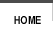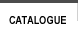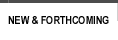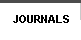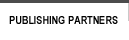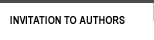sitemap | contact usISBN Author Title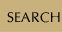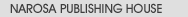Book SeriesJournalsBook Proposal FormWriting a BookUsing Published MaterialRights and PermissionsExamination Copies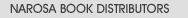List of PublishersBargains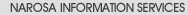Services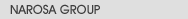About NarosaHistoryMissionGroup CompaniesOur StrengthAlliances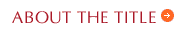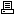view in print modeIntroduction to Differential Equations using MATLAB, An Author(s): Rizwan Butt ISBN:    978-81-8487-515-7  E-ISBN:    Publication Year:   2016 Pages:   592 Binding:   Paper Back Dimension:   185mm x 240mm Weight:   950 Textbook

 About the book An Introduction to Differential Equations using MATLAB exploits the symbolic, numerical, and graphical capabilities of MATLAB to develop a thorough understanding of differential equations algorithms. This book provides the reader with numerous applications, m-files, and practical examples to problems. Balancing theoretical concepts with computational speed and accuracy, the book includes numerous short programs in MATLAB that can be used to solve problems involving first-and higher-order differential equations, Laplace transforms, linear systems of differential equations, numerical solutions of differential equations, computer graphics, and more. The author emphasizes the basic ideas of analytical and numerical techniques and the uses of modern mathematical software (MATLAB) rather than relying only on complex mathematical derivations to engineers, mathematician, computer scientists, and physicists or for use as a textbook in applied or computational courses. A CD-ROM with all the figures, codes, solutions, appendices, an introduction to MATLAB commands, and m-files for all of the programs is available on request. Table of Contents First-Order Differential Equations / Higher-Order Linear Differential Equations / Laplace Transforms / Linear Systems of Differential Equations / Series Solutions of Differential Equations / Numerical Solutions of Differential Equations / Partial Differential Equations / Index. Audience Under  Postgraduate Students & Researchers

# var message="For Bargains from Narosa Book Distributors, " var neonbasecolor="black" var neontextcolor="red" var neontextcolor2="red" var flashspeed=100 // speed of flashing in milliseconds var flashingletters=2 // number of letters flashing in neontextcolor var flashingletters2=2 // number of letters flashing in neontextcolor2 (0 to disable) var flashpause=0 // the pause between flash-cycles in milliseconds ///No need to edit below this line///// var n=0 if (document.all||document.getElementById){ document.write('<font color="'+neonbasecolor+'" size="5">') for (m=0;m<message.length;m++) document.write('<span id="neonlight'+m+'">'+message.charAt(m)+'</span>') document.write('</font>') } else document.write(message) function crossref(number){ var crossobj=document.all? eval("document.all.neonlight"+number) : document.getElementById("neonlight"+number) return crossobj } function neon(){ //Change all letters to base color if (n==0){ for (m=0;m<message.length;m++) crossref(m).style.color=neonbasecolor } //cycle through and change individual letters to neon color crossref(n).style.color=neontextcolor if (n>flashingletters-1) crossref(n-flashingletters).style.color=neontextcolor2 if (n>(flashingletters+flashingletters2)-1) crossref(n-flashingletters-flashingletters2).style.color=neonbasecolor if (n<message.length-1) n++ else{ n=0 clearInterval(flashing) setTimeout("beginneon()",flashpause) return } } function beginneon(){ if (document.all||document.getElementById) flashing=setInterval("neon()",flashspeed) } beginneon() CLICK HERE

Group
| Companies | Mission | Strength | Values | History | Contact us